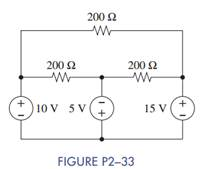### Create an Account

Already have account?

### Forgot Your Password ?

Home / Questions / In Figure P2−33 a Assign a voltage and current variable to every element b Use KVL to find...

# In Figure P2−33 a Assign a voltage and current variable to every element b Use KVL to find the voltage across each resistor Use Ohm’s law to find the current through each resistor

In Figure P2−33:

(a) Assign a voltage and current variable to every element.

(b) Use KVL to find the voltage across each resistor.

(c) Use Ohm’s law to find the current through each resistor.

(d) Use KCL to find the current through each voltage source.Jun 15 2020 View more View Less

#### Answer (Solved)Subscribe To Get Solution